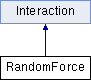ParM  parm A molecular dynamics library
RandomForce Class Reference

#include <interaction.hpp>

Inheritance diagram for RandomForce:Public Member Functions

RandomForce ()

RandomForce (AtomGroup &agroup, flt force_mag, flt freq, RandomForceType force_type=UNIFORM)

uint size () const

RandomForceAtom get (uint i) const

bool add (RandomForceAtom a, bool replace=true)
If "replace", a previous pair found will be replaced by the new pair. More...

flt energy (Box &box)
Potential energy due to this Interaction. More...

void set_forces (Box &box)

flt pressure (Box &box)
Partial pressure due to this Interaction. More...

flt set_forces_get_pressure (Box &box)
Set forces (Atom.f) and return $$P = \sum_{\left<i,j \right>} \vec r_{ij} \cdot \vec F_{ij}$$ at the same time (see pressure()). More...Public Member Functions inherited from Interaction
virtual Matrix stress (Box &box)
The force-moment tensor for the current simulation: More...

virtual ~Interaction ()

Public Attributes

vector< RandomForceAtomgroup

Constructor & Destructor Documentation

 RandomForce::RandomForce ( )
inline
 RandomForce::RandomForce ( AtomGroup & agroup, flt force_mag, flt freq, RandomForceType force_type = UNIFORM )
inline

Member Function Documentation

 bool RandomForce::add ( RandomForceAtom a, bool replace = true )

If "replace", a previous pair found will be replaced by the new pair.

If not "replace" and that pair of atoms is already inserted, an error will be thrown.

 flt RandomForce::energy ( Box & box )
inlinevirtual

Potential energy due to this Interaction.

Implements Interaction.

 RandomForceAtom RandomForce::get ( uint i ) const
inline
 flt RandomForce::pressure ( Box & box )
inlinevirtual

Partial pressure due to this Interaction.

$$P = \sum_{\left<i,j \right>} \vec r_{ij} \cdot \vec F_{ij}$$, or equivalently $$P = \sum_i \vec r_i \cdot \vec F_i$$

Note that the full pressure involves all interactions and temperature, and needs to be normalized by $$\frac{1}{dV}$$ where $$d$$ is the number of dimensions and $$V$$ is the volume.

Implements Interaction.

 void RandomForce::set_forces ( Box & box )
virtual

Implements Interaction.

 flt RandomForce::set_forces_get_pressure ( Box & box )
inlinevirtual

Set forces (Atom.f) and return $$P = \sum_{\left<i,j \right>} \vec r_{ij} \cdot \vec F_{ij}$$ at the same time (see pressure()).

Reimplemented from Interaction.

 uint RandomForce::size ( ) const
inline

Member Data Documentation

 vector RandomForce::group

The documentation for this class was generated from the following files: